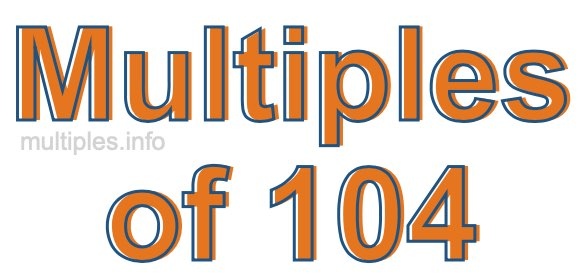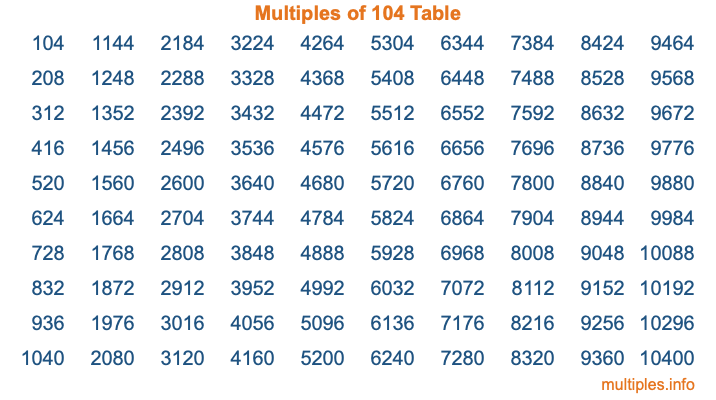Multiples of 104Welcome to the Multiples of 104 page. Here we will first teach you everything you will ever need to know about the multiples of 104, and then give you a study guide summary of everything we taught you to make sure you remember it all. Use this page to look up facts and learn information about the multiples of 104. This page will make you a multiples of one hundred four expert!

Definition of Multiples of 104
Multiples of 104 are all the numbers that when divided by 104 equal an integer. Each of the multiples of 104 are called a multiple. A multiple of 104 is created by multiplying 104 by an integer.

Therefore, to create a list of multiples of 104, you start with 1 multiplied by 104, then 2 multiplied by 104, then 3 multiplied by 104, and so on for as long as you want. Thus, the list of the first five multiples of 104 is 104, 208, 312, 416, and 520. To see a larger list of multiples of 104, see the printable image of Multiples of 104 further down on this page. We also have a category where you can choose any nth multiple of 104.

Multiples of 104 Checker
The Multiples of 104 Checker below checks to see if any number of your choice is a multiple of 104. In other words, it checks to see if there is any number (integer) that when multiplied by 104 will equal your number. To do that, we divide your number by 104. If the the quotient is an integer, then your number is a multiple of 104.

Is  a multiple of 104?

Least Common Multiple of 104 and ...
A Least Common Multiple (LCM) is the lowest multiple that two or more numbers have in common. This is also called the smallest common multiple or lowest common multiple and is useful to know when you are adding our subtracting fractions. Enter one or more numbers below (104 is already entered) to find the LCM.

Check out our LCM Calculator if you need more details about the Least Common Multiple or if you need the LCM for different numbers for adding and subtraction fractions.

nth Multiple of 104
As we stated above, 104 is the first multiple of 104, 208 is the second multiple of 104, 312 is the third multiple of 104, and so on. Enter a number below to find the nth multiple of 104.

th multiple of 104

Multiples of 104 vs Factors of 104
104 is a multiple of 104 and a factor of 104, but that is where the similarities end. All postive multiples of 104 are 104 or greater than 104. All positive factors of 104 are 104 or less than 104.

Below is the beginning list of multiples of 104 and the factors of 104 so you can compare:

Multiples of 104: 104, 208, 312, 416, 520, etc.

Factors of 104: 1, 2, 4, 8, 13, 26, 52, 104

As you can see, the multiples of 104 are all the numbers that you can divide by 104 to get a whole number. The factors of 104, on the other hand, are all the whole numbers that you can multiply by another whole number to get 104.

It's also interesting to note that if a number (x) is a factor of 104, then 104 will also be a multiple of that number (x).

Multiples of 104 vs Divisors of 104
The divisors of 104 are all the integers that 104 can be divided by evenly. Below is a list of the divisors of 104.

Divisors of 104: 1, 2, 4, 8, 13, 26, 52, 104

The interesting thing to note here is that if you take any multiple of 104 and divide it by a divisor of 104, you will see that the quotient is an integer.

Multiples of 104 Table
Below is an image of the first 100 multiples of 104 in a table. The table is in chronological order, column by column. The first column has the first ten multiples of 104, the second column has the next ten multiples of 104, and so on.The Multiples of 104 Table is also referred to as the 104 Times Table or Times Table of 104. You are welcome to print out our table for your studies.

Negative Multiples of 104
Although not often discussed or needed in math, it is worth mentioning that you can make a list of negative multiples of 104 by multiplying 104 by -1, then by -2, then by -3, and so on, to get the following list of negative multiples of 104:

-104, -208, -312, -416, -520, etc.

Multiples of 104 Summary
Below is a summary of important Multiples of 104 facts that we have discussed on this page. To retain the knowledge on this page, we recommend that you read through the summary and explain to yourself or a study partner why they hold true.

There are an infinite number of multiples of 104.

A multiple of 104 divided by 104 will equal a whole number.

104 divided by a factor of 104 equals a divisor of 104.

The nth multiple of 104 is n times 104.

The largest factor of 104 is equal to the first positive multiple of 104.

104 is a multiple of every factor of 104.

104 is a multiple of 104.

A multiple of 104 divided by a divisor of 104 equals an integer.

104 divided by a divisor of 104 equals a factor of 104.

Any integer times 104 will equal a multiple of 104.

Multiples of a Number
Here you can get the multiples of another number, all with the same attention to detail as we did for multiples of 104 on this page.

Multiples of
Multiples of 105
Did you find our page about multiples of one hundred four educational? Do you want more knowledge? Check out the multiples of the next number on our list!